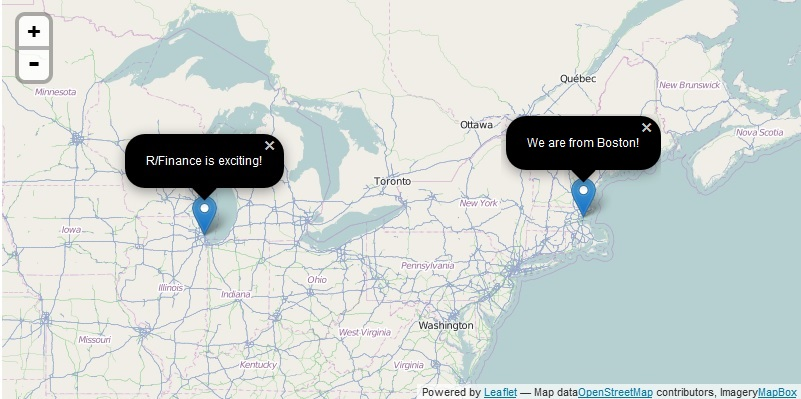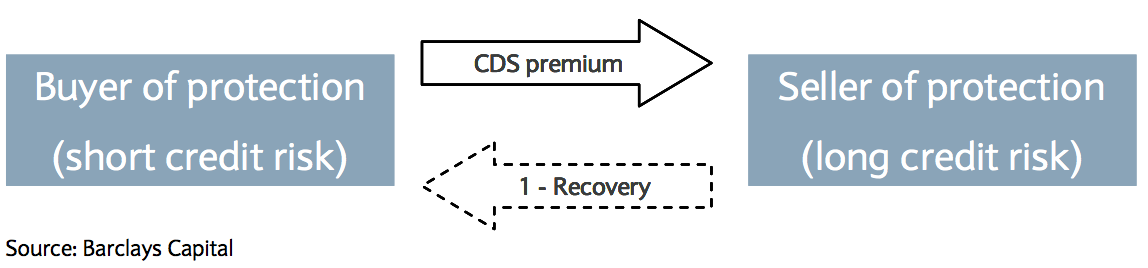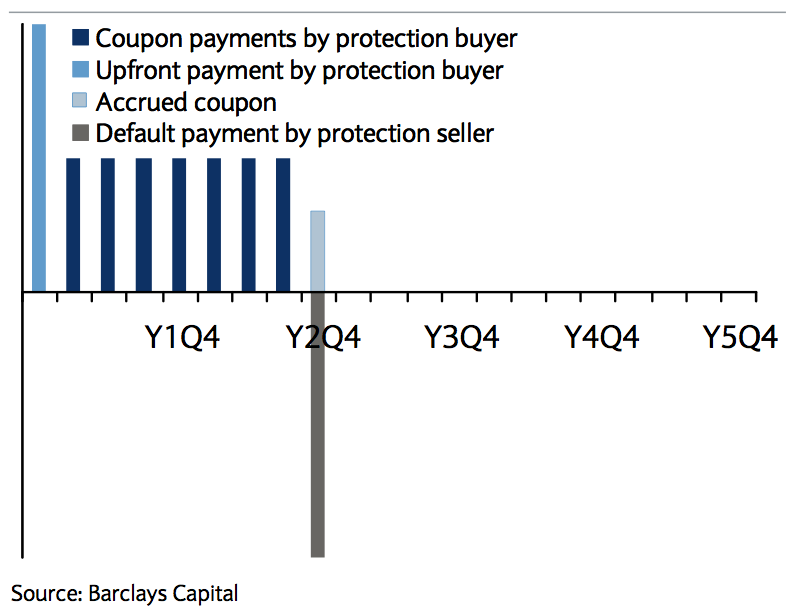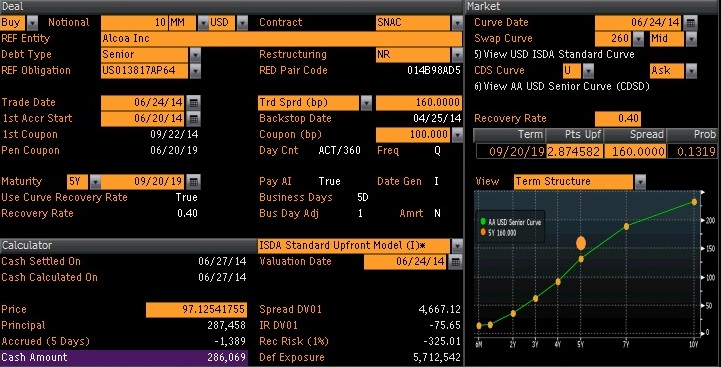Wednesday, May 27, 2015

## Who are we?

### Yuanchu and Zijie## David Kane wants to say Hi!## CDS Basics## CDS Basics## General Introduction to creditr

```## not run
library(devtools)
install_github("yuanchu/creditr")
library(creditr)```

## General Introduction to creditr

S4 design: CDS class

```library(creditr)
x <- CDS(date   = as.Date("2014-05-07"),
tenor  = 5,
coupon = 100)
slotNames(x)```
```##   "name"        "contract"    "RED"         "date"        "spread"
##   "maturity"    "tenor"       "coupon"      "recovery"    "currency"
##  "notional"    "principal"   "accrual"     "pd"          "price"
##  "upfront"     "spread.DV01" "IR.DV01"     "rec.risk.01"```

## Main Functionalities

• Spread, upfront, and default probability.

• Exposure measurements.

• Real time data and other supportive layers.

## Spread, upfront and default probability

```spread.to.upfront(data.frame(date     = Sys.Date(),
tenor    = 5,
recovery = 0.4,
coupon   = 100,
currency = "USD"))```
`##  259021`

## Spread, upfront and default probability

```upfront.to.spread(data.frame(date     = Sys.Date(),
upfront  = 1000000,
tenor    = 5,
recovery = 0.4,
coupon   = 100,
currency = "USD"))```
`##  335.7157`

## Spread, upfront and default probability

```spread.to.pd(data.frame(date     = Sys.Date(),
tenor    = 5,
recovery = 0.4,
currency = "USD"))```
`##  0.1279975`

## Spread, upfront and default probability

```pd.to.spread(data.frame(date     = Sys.Date(),
pd       = 0.1,
tenor    = 5,
recovery = 0.4,
currency = "USD"))```
`##  123.0821`

## Sensitivity to change in spread

```# calculates the change in upfront value when the spread rises
# by 1 basis point.

x <- data.frame(date     = as.Date(c("2014-04-22", "2014-04-22")),
currency = c("USD", "EUR"),
tenor    = c(5, 5),
coupon   = c(100, 100),
recovery = c(0.4, 0.4),
notional = c(1e7, 1e7))

`##  4768.084 4878.017`

## Sensitivity to change in spread

\(CS10\)

```# calculates the change in upfront value when the spread rises 10%

CS10(x)```
`##  56952.65 53430.42`

## Exposure to interest rate

\(IR.DV01\)

```# calculate the change in upfront when there is a 1 basis point
# increase in interest rate

IR.DV01(x)```
`##  -25.15333 -12.85976`

## Exposure to recovery rate

\(rec.risk.01\)

```# calculates the amount of change in upfront when there is a 1%
# increase in recovery rate

rec.risk.01(x)```
`##  -82.18602 -38.82727`

## Real time data

• Dates and conventions.

## Interfaces

### Functions are awesome for quants, but what if I …

• am new to `R`?

• just want a result from a beautiful interface?

• want a play toy when reading a introduction paper of CDS?

## Bloomberg Terminal

### Terminology is intimidating! And it's not free!